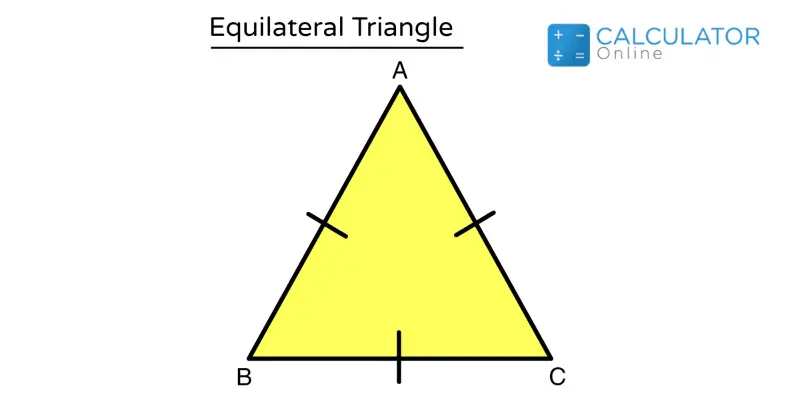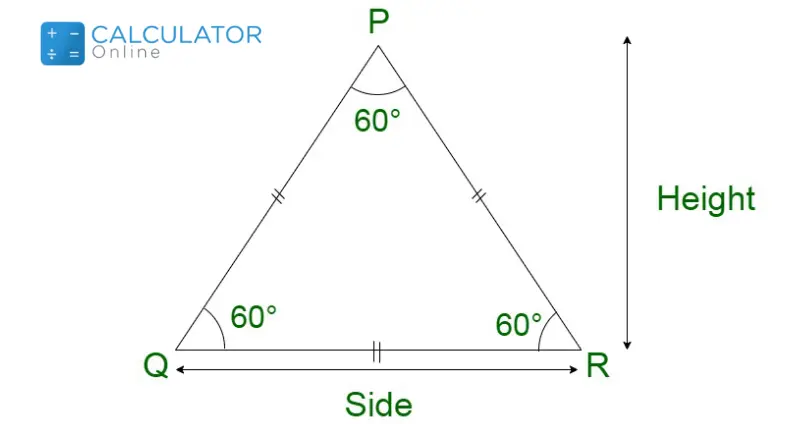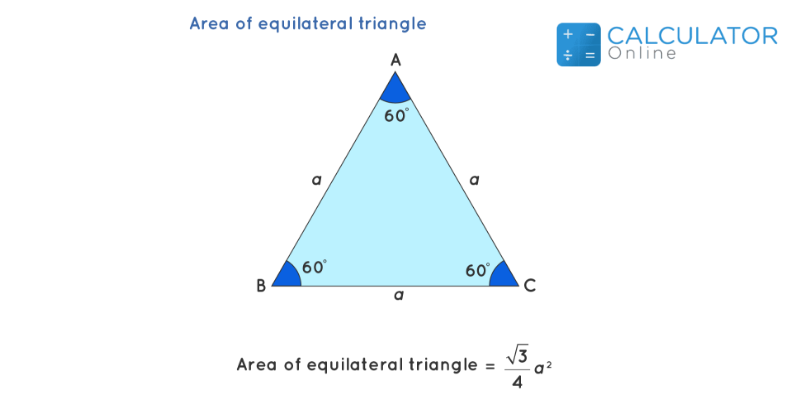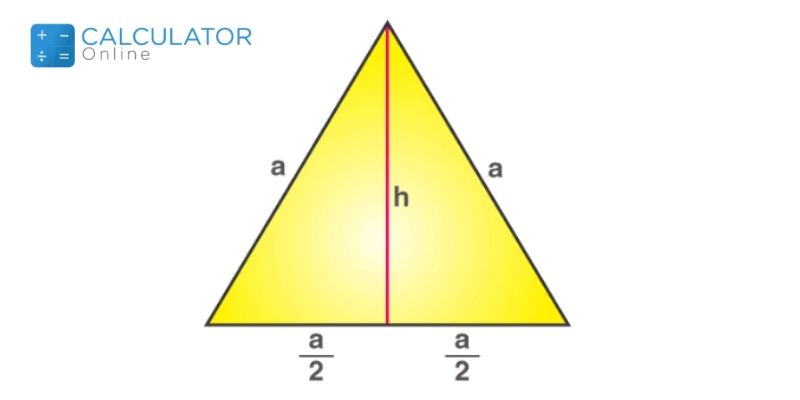Uh Oh! It seems you’re using an Ad blocker!

We always struggled to serve you with the best online calculations, thus, there's a humble request to either disable the AD blocker or go with premium plans to use the AD-Free version for calculators.

Or# Equilateral Triangle Calculator

Choose a Calculation:

K:

Table of Content

Get the Widget!

Add this calculator to your site and lets users to perform easy calculations.

Feedback

How easy was it to use our calculator? Did you face any problem, tell us!

The equilateral triangle calculator assists you in the calculation of the standard parameters of an equilateral triangle. The standard parameter can be the area, height, and perimeter.

## Equilateral Triangle:

The Equilateral Triangle is a special case of a triangle having all the slides equal to each and also the angles.

An equilateral triangle is also known as a regular triangle, there are certain properties an equilateral triangle has:• The equilateral triangle has all the sides equal to each other.

Sides of Equilateral Triangle: a = b = c

• The equilateral triangle measurements of angles equal 60°, and these angles are congruent with each other.

$$m∠A = m∠B = m∠C = 60^\text{o}$$

• The altitude, the angle bisector, the perpendicular bisector, and the median of the equilateral triangle coincide with each other

### Equilateral Triangle Formula For Area:

The Equilateral triangle formula for Area can be derived by two following methods:

It depends on how you are going to find the area and what factors you have in the equilateral triangle equation.

#### Case 1:

Consider if you have the Base and Height of the  Equilateral triangle, then you can find the area by the given formula:

$$Area = \frac{1}{2} Base * Height$$#### Case 2:

Consider you know the length of the sides of the Equilateral triangle, then use the equilateral triangle formula:

$$Area=\dfrac{\left(a^{2}*\sqrt{3}\right)}{4}$$### How to Find the Height of an Equilateral Triangle?

You can calculate the height by the height of the equilateral triangle formula:

$$Height=\dfrac{\left(Side*\sqrt{3}\right)}{2}$$### Equilateral Triangle Measurements For Perimeter:

The equilateral triangle equations for various measurements of the Perimeter are given below:

• The perimeter of Equilateral Triangle: P = 3a

The equilateral triangle formula for Semiperimeter:

• Semiperimeter of Equilateral Triangle: s = 3a / 2

#### Example 1:

Calculate height of equilateral triangle and its area whose side is 4cm.

Solution:

The length side  “a” = 4cm

We know that the area of the equilateral triangle is (√3/4)a2

Now, substitute the value a = 4 in the formula

A = (√3/4)42

A = √3 (4)

A = 1.732 (4)

A = 6.928 cm2

h = a × √3 / 2

h =  4 × √3 / 2

h =  4 × 0.866

h = 3.464 cm

Perimeter =  P = 3a

Perimeter =  P = 3(4)

Perimeter =  P = 12cm

Semiperimeter: s = 3a / 2

Semiperimeter: s = 12 / 2

Semiperimeter: s = 6 cm

You can measure the area, height, perimeter, and semi-perimeter with the equilateral triangle calculator.

### How to Use the Equilateral Triangle Calculator?

For various measurements possibilities, just follow the instructions given below:

Input:

• Select the parameters from the list
• Enter the required value of the parameter
• Hit the calculate Button

Output:

• The Side length
• The Area and Height
• The Perimeter and Semiperimeter

### References:

From the source of Wikipedia: Equilateral Triangle, Principal properties

From the source of study.com: Triangles, Angles